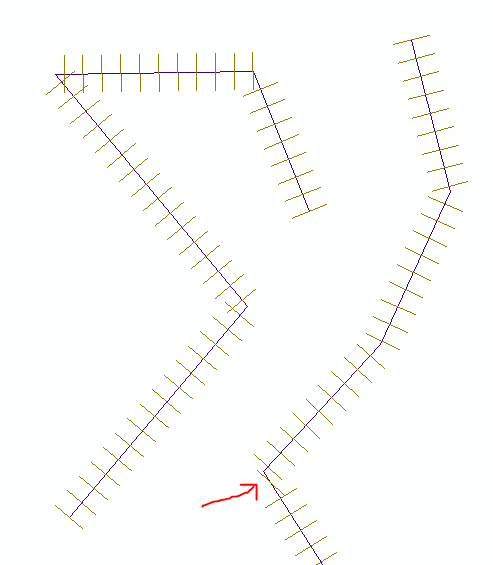# Creating many line features with python script

8161
10
07-30-2015 10:41 AM
Labels (3)
Highlighted

I am currently analyzing a point density map from data taken over a mountainous area in ArcMap. I have a line feature that I made expressing a flight path. I would like to draw a bunch of lines perpendicular to this flight path. Using the feature construction tool, I was able to draw a few of these lines. I then used Stack Profile in the 3D Analyst toolbox so that I could find the point densities in relation to the distance away from the initial vertex. However, for the purpose of my analysis, I would like to have many lines (~100) evenly from the beginning to the end of the flight path line spanning the entire swath of the data set for several point density maps.

As a result, manually creating these lines would be very tedious so I was hoping to be able to create a python script to speed up the process and be able to easily do this for future analyses. How will I be able to do this with a python script, if it is possible? I have very little experience with python scripts, so any help on this is greatly appreciated.

Tags (3)
10 Replies
Highlighted
byMVP Honored Contributor

An easy way to do this is to use ET GeoWizards Create Station Lines tool (one example here).

Aside from that, this is definitely possible using arcpy geometries, but it will take some tedious trig coding.

Highlighted

I cannot get access to ET GeoWizards. Do you know how I can do the tedious trig coding?

Highlighted
byMVP Esteemed Contributor

I don't have time right now to help with the python (although it is an interesting and doable script), but conceptually, one thing you could do to get the points and parallel lines is to create a copy of the line (offlset), then move alone the parallel line creating points at you given distance, then use the near distance.    (many apologies for not even looking up the proper python commands...but sometimes getting the concept worked out helps....then the python is much easier)

Highlighted
byMVP Honored Contributor

Here's a script that should get you most of the way there. There is some issue with indexing, thinking it's still on the previous line segment, but I'll leave that for you to sort out.

`... perpLines = []... fc = "line"... sr = arcpy.Describe(fc).spatialReference... perpLineSpacing = 1000... perpLineLength = 1000... with arcpy.da.SearchCursor(fc,"SHAPE@",spatial_reference=sr) as cursor:...     for row in cursor: ...         for part in row: # part = a line array...             for i in range(len(part)):...                 if i==0: # first vertex...                     perpLineCounter = 0...                 else:...                     dy = part.Y - part[i-1].Y...                     dx = part.X - part[i-1].X...                     segmentAngle = math.degrees(math.atan2(dy,dx))...                     segmentLength = math.sqrt(math.pow(dy,2)+math.pow(dx,2))...                     linesOnSegment = int(segmentLength/perpLineSpacing)...                     for line in range(linesOnSegment+1):...                         point = row.positionAlongLine(perpLineCounter * perpLineSpacing)...                         left = arcpy.Point(point.centroid.X - (math.cos(math.radians(segmentAngle-90))*perpLineLength), point.centroid.Y - (math.sin(math.radians(segmentAngle-90))*perpLineLength))...                         right = arcpy.Point(point.centroid.X + (math.cos(math.radians(segmentAngle-90))*perpLineLength), point.centroid.Y + (math.sin(math.radians(segmentAngle-90))*perpLineLength))...                         perpLines.append(arcpy.Polyline(arcpy.Array([left,right]),sr))...                         perpLineCounter += 1... arcpy.CopyFeatures_management(perpLines ,r'in_memory\lines')`Highlighted

Awesome! I'll see what I can do with that. Thank you very much!!

Highlighted
New Contributor II

I would like to do the same operation only create parallel lines, not perpendicular.  How would I modify the script to do this?  What would the math equations change to?  Thanks for your help.

-Jason

Highlighted
byMVP Esteemed Contributor

only got a few?

they aren't all the same in terms of spacing and ends?

any other variants?

do you want the copy to maintain the X or Y min/max?

For full control and for one offs so you can play around, make a copy, get into an edit session and ...Copying parallel line features—Help | ArcGIS for Desktop

Highlighted
New Contributor II

Thanks for the quick response Dan.  I have been using the create parallel tool during a edit session for my current use.  However, I'd like a python script to avoid having to do this manually every time because sometimes I need to create a lot of parallel lines.

Basically I need to create parallel lines/transects from a road centerline for our survey crews.  The road centerline would be input #1, the spacing between transects/parallel lines would be input #2, and the total number of transects/parallel lines to create would be input #3 in the tool.

Thanks again,

Jason

Highlighted
byMVP Honored Contributor

If you can tolerate the line ends being connected together, you can explore Buffer (probably want to run in batch mode, and specify flat line end type) or Multiple Ring Buffer. Then, convert to line.